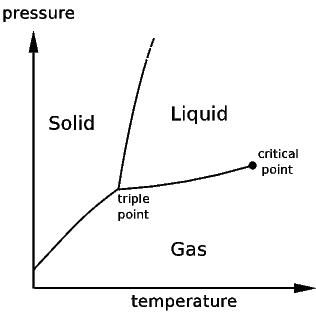Degrees of Freedom

Consider again an aluminum atom. The atom individually can be described by a few numbers representing its speed, its position, and the nature of its internal structure. Scientists have been able to very accurately account for all the properties of single atoms in sensitive experiments. To accurately predict and understand such experiments, these numbers must all be measured very carefully. This is not so difficult when there are only a few atoms, but in any material the number of atoms under consideration is astronomical. The number of atoms in a material is given in terms of Avogadro's number, which is 6.022E23.

Thus, for a solid which consists of so many atoms together, it would seem as though an uncountable number of measurements would be necessary to account for all the atoms, as they each possess the aforementioned qualities independently. For a can of aluminum, which contains tens of 1023 atoms, it is staggering that anyone could describe the state of the material. This is the problem of having many degrees of freedom, and when those degrees of freedom are intertwined in a non-negligible way, problems in many body physics arise.

The state of a system can be described in terms of its degrees of freedom. For example, a gas of oxygen atoms (the system), can be described by the positions and velocities of every atom. However, for any macroscopic quantity of gas where there are on the order of 1023 particles, keeping track of that many numbers would make for an impossibly difficult calculation. Rather remarkably, just like the atom, the can be described by only a few numbers. Thus, the number of thermodynamic degrees of freedom is quite few. Common thermodynamic quantities include the temperature, pressure, density, refractive index, and compressibility of a material. Every class of materials can be described by its own list of thermodynamic variables and while the full list may not be short, it is certainly not 1023 long!

A phase transition is achieved by changing the thermodynamic parameters to reach a particular limit. For example, the boiling of water will occur at one hundred degrees Celsius, but this is only at a particular pressure. At a lower pressure the water would boil at a lower temperature. Thus, the boiling of water or any liquid can be defined by a curve in the parameter space of pressure and temperature. That is, there is a function T(P) that gives the temperature at which water will boil at a particular ambient pressure. This is called a phase diagram, and when many phases are available, there will be many curves in the parameter space to distinguish which phase will be exhibited at those parameters values. Additionally, other thermodynamic variables besides temperature and pressure are used to describe the boundaries between phases. In some systems a pressure is not even defined, or the transition may be independent of pressure entirely. In the case of diblock copolymers which are effectively incompressible (and thus have properties that are independent of pressure), the relevant thermodynamic parameters are the so-called Flory parameter describing the interactions between monomer sub chains, and the bulk fraction of one monomer versus another. The phase transitions are only dependent on temperature implicitly through the Flory parameter.This shows qualitatively a very simple phase diagram for a given material. The liquid, solid, and gas phases are separated by curves in the T,P parameter space. On these curves, both phases are represented to some degree simultaneously.A slightly more exotic phase transition, this diagram shows the phases of a binary alloy in a parameter space defined by the bulk fraction of one metal and the temperature.
 Previous Home Next